Courses

# Setting & Sedimentation - 2 Computer Science Engineering (CSE) Notes | EduRev

## Electronics and Communication Engineering (ECE) : Setting & Sedimentation - 2 Computer Science Engineering (CSE) Notes | EduRev

The document Setting & Sedimentation - 2 Computer Science Engineering (CSE) Notes | EduRev is a part of Electronics and Communication Engineering (ECE) category.
All you need of Electronics and Communication Engineering (ECE) at this link: Electronics and Communication Engineering (ECE)

TYPES OF GRAVITATIONAL SETTLING PHENOMENON

(i) Discrete particle settling: Applicable for very low concentration solids

• Particles settle as individual entities
• No interaction between particles

(ii) Flocculation settling: Applicable for dilute suspension of particles that coalesce or flocculate

• By flocculation, particle size increases and terminal velocity increases.
• Settling can be increased by addition of some ballasting agent such as polymers.

(iii) Hindered settling

•  For suspension of intermediate settling.
• In this case, particles are such close together that the inter-particle force due to one hinders the settling of other particle.
• The particles remain in fixed position with respect to each other and particles settles as a whole

(iv) Compression settling

• Case in which particles are in such high concentration that a whole structure is formed.
• Compression takes place due to weight of whole mass which continuously increases.
• A clear water is formed above compression zone

CLASSIFICATION OF SEDIMENTATION TANKS

• Grit chamber: For removal of sand, grits, etc.
• Plain sedimentation tank: For removal of settleable solids.
• Chemical precipitation tank: for removal of very fine suspended particles by adding coagulants, etc
• Septic tanks: For doing sedimentation and sludge digestion together in households
• Secondary settling tanks: After activated sludge or trickling filter treatment systems.

SCOUR VELOCITY

Maximum horizontal velocity though the tank which does not allows resuspension (scouring) of settled particles. It is given as :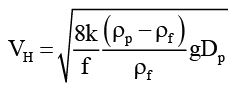(3.8.1)
Where, f is the Darcy–Weisbach friction factor (unit-less) and its value varies in the range 0.02- 0.03; k is cohesion constant that depends upon the type of material being scoured (unit-less). Its value varies in the range of 0.04- 0.06. For sticky interlocking matter k=0.6 whereas for ungrounded sand k=0.4.

Important point in design of sedimentation tank  Assume t is the detention time for which a suspension is detained in the settling tank having height H, length L and width W. Also assume, VH is the horizontal velocity and ut is the terminal settling velocity of the target particle. Now, Cross-sectional area of tank (AC)=H×W Surface area of tank (A)=L×W If Q is the flow rate of wastewater into the tank,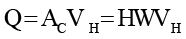(3.8.2)
Since the target particle should not re-suspend during its flow along the length of the tank, therefore, detention time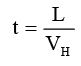(3.8.3)
Also, the target particle should settle down before it reaches the outlet, therefore,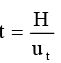(3.8.4)
Combining,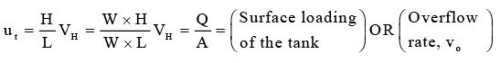(3.8.5)

This expression gives following important points:

• The terminal velocity should be ≥ surface loading of the tank.
• Surface area is more important than the height of the settling tank.
• Higher the surface area, higher will be the removal efficiency and more will be the removal of finer particles.
• All particles having settling velocity ut ≥ vo will be completed removed.
• For particles having ut < vo, only ut/vo only fraction will be removed.

Problem 3.8.1: A municipal wastewater plant is to be designed to treat maximum flow rate of 60000 m3/d. Target particle for settling has the following characteristics: DP=200×10-6 m, k=0.05, f=0.025, ρP=1.25×103 kg/m3. For a rectangular classifier having ratio of length to width>6, overflow rate is at-least four times the settling velocity and horizontal velocity at-most one-third of the scour velocity.  (a) Find the dimensions of the rectangular tank  (b) Determine detention time

Solution: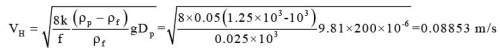Actual horizontal velocity=VH/3=0.02951 m/s.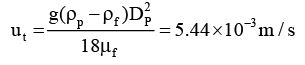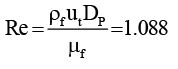Overflow rate=3×u= 21 .7 6 x 10 -3 m / s

If W is the width, L is the length and H is the height of the rectangular settling basin,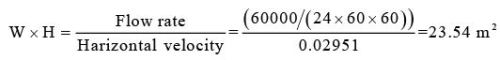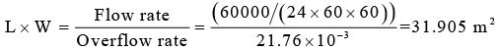Also given: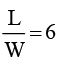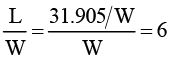W=2.305 m,
L=6×2.305=13.83 m
H=23.54/2.305=10.21 m
Volume of tank, V=LWH=325.47 m3

Detention time,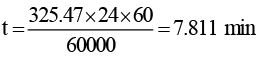Offer running on EduRev: Apply code STAYHOME200 to get INR 200 off on our premium plan EduRev Infinity!

,

,

,

,

,

,

,

,

,

,

,

,

,

,

,

,

,

,

,

,

,

;